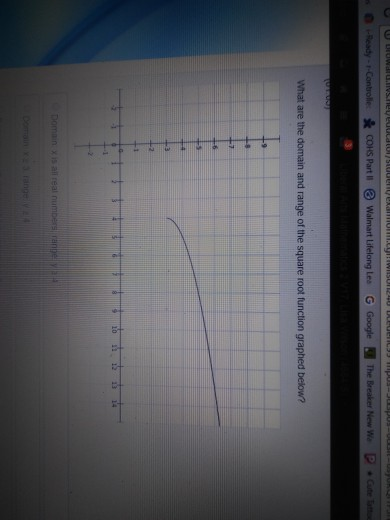# What is the domain of the square root function graphed belowWhat are the domain and range of the squareroot function graphed below? Domain x is all real numbers range y = 1 Domain x = 3 range y = 4

Here from graph it is clear that Domain of the function
( Look at X-axis and graph) starts form 4 onwords and it
continues
Therefore Domain of f =
Now look at Y-axis and graph , It is clear that Range
starts form y=3 and it increases
Therefore Range
Domain   and